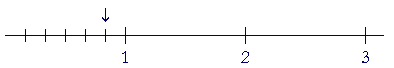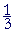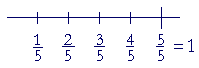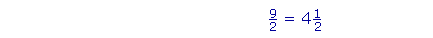S k i l l
i n
A R I T H M E T I C

Lesson 20

# WHAT IS A FRACTION?

## COMMON FRACTIONSPROPER FRACTIONSMIXED NUMBERSIMPROPER FRACTIONSA fraction is a number we need for measuring. For counting, we have the natural numbers: 1, 2, 3, 4. But when we measure something, such as a length, it will not always be a whole number. Therefore we need numbers that are less than 1 -- numbers that are the parts of 1: half of 1, a third, a fourth, a fifth, a millionth.

Why those ordinal numbers: third, fourth, fifth? That is how language itself names which part (Lesson 15); that use of ordinals is logically and historically prior to the names of fractions.The number we call "one-third," for example, refers to a specific point on the number line. It marks and names the third part of number 1.

What is usually taught as fractions:13 means 1 out of 3

is actually parts, taught with fractional symbols. But a mathematical fraction is not simply a part of anything. It is a part of the unit of measure, which is 1. 1 is now a continuous unit.

 1. What is a common fraction? A number written with a numerator and a denominator, in which both are natural numbers.

Example 1.("three-tenths") is a common fraction.  It is in contrast to .3, which is a decimal fraction, or simply a decimal.

The numerator ofis 3.  The denominator is 10.  They are called the terms of the fraction.

 2. What do the denominator and the numerator signify? The denominator names the number of equal parts into which number 1 has been divided. We read the denominator as an ordinal number. It names which part of number 1.The numerator, which we read as a cardinal number, names how many of those parts.The fractionsignifies that number 1 has been divided into 10 equal parts and -- starting from the left -- we are counting 3 of them.

Number 1, in other words, has been divided into tenths.  At this point, the student should be clear about the language of division into equal parts, and why we use ordinal numbers:  third, fourth, fifth, sixth, and so on.  See Lesson 3, the topic Division into equal parts.

Every fraction is constructed in this way from number 1, which is the source of every number of arithmetic. The whole numbers are the multiples of 1. The fractions are its parts:  its halves, thirds, fourths, fifths, and so on.

Since the numerator and denominator are natural numbers, the numerator has a ratio to the denominator. (3 is three tenths of 10.) And the fraction itself has that same ratio to 1. (3/10 is three tenths of 1.) .

Fractional symbols therefore indicate a ratio. The fractionindicates the ratio of 1 to 2. 1 is half of 2.

A proportion can then be written as

 12 = 48 .

"1 is to 2 as 4 is to 8."

Any number that can be written as a fraction is therefore called a rational number

Now in everyday speech, a fraction means a part of a whole, as in the phrase "a fraction of the students." But students are discrete not continuous, and in mathematics a fraction is a number we need to measure what is continuous. A mathematical fraction is not simply a part of any whole. It is a part of the unit of measure, which is 1. As for a pie divided into equal parts, it can and should be described verbally -- not with fractions. We do not measure pies. We will go into this more below.

Example 2.   If number 1 is divided into 5 equal parts, and we count 4 of them, what fraction is that?  Also, into which parts has number 1 been divided?

 Answer.   That fraction is 45 ("Four-fifths").Number 1 has been divided into fifths.

Note:  To divide number 1 into fifths, we cut the line four times. We cut the line one less than the name of the part.

In the next Lesson we will see that number 1 has been decomposed into unit fractions, which are fractions whose numerator is 1.Every fraction is thus a number -- a sum -- of unit fractions. Addition and subtraction, whether with whole numbers or fractions, are done with a number of units.

3.   What is a proper fraction?

 23
A fraction that is less than 1.

4.   How can we recognize a proper fraction?

 23
The numerator is smaller than the denominator.("two-thirds") is a proper fraction.  It is less than 1.On the number line, it falls to the left of 1.  Specifically,is two thirds of 1, as we will see presently.

5.   In English, how are the proper fractions named?

 12

Since the numerator and denominator are natural numbers, they have a ratio to one another. And a proper fraction has the same name as that ratio.

Example 3.   The number we write as 1 over 2 ---- is called "one-half" because of the ratio of 1 to 2.  1 is one half of 2.  And thefraction itself is one half of 1.

Notice that we write the name of the fraction hyphenated, but the name of the ratio unhyphenated. In that way we maintain the distinction between fractions and ratios. See below.

Example 4.   Why is this numbercalled "two-thirds"?

Answer.  Because of the ratio of  2 to 3.  2 is two thirds of 3.  And on the number line, that fraction is two thirds of 1.To placeon the number line, we must cut the length representing 1 into thirds, that is, into three equal parts.  We cut it only twice.

"One third, two thirds."

Example 5.   This number  ¼  is called "one-quarter" or "one-fourth," because the numerator is one quarter of the denominator -- and ¼ itself is one quarter of 1.To place ¼ on the number line, cut the line one less than the name of the part.  To divide 1 into fourths, cut the line three times.

Example 6.   Read these numbers:"Five-eighths""Sixteen-thirds" The improper fractions (see below) are named like the proper fractions."Nine-halves" (Not "Nine 2's.")"27 over 39" With large numbers, it is not necessary to say "27 thirty-ninths.""56 thousandths" When the denominator is a power of 10, however, we always say the decimal name.  (Lesson 3)"5 and 79 hundredths" This is a mixed number; see below.

Example 7.   What number is at the arrow?Answer.  Number 1 has been cut five times -- into six equal parts. That

 number is 56 .

The proper fractions are the parts of 1.

 6. What is a mixed number?A whole number plus a proper fraction.

Example 8.-- "2 and one-third" -- is a mixed number.  It is 2 plus.

The and in "2 and one-third" means plus.To placeon the number line, the unit between 2 and 3 must be cut into thirds.  We cut the line twice.

Improper fractions

If we divide each whole unit into thirds, say, and keep counting them -- then we will come to,,, and so on.  That is, we will come to fractions that are equal to or greater than 1.  We call those improper fractions.

 7. What is an improper fraction?A fraction greater than or equal to 1.

We can recognize an improper fraction when the numerator is greater than or equal to the denominator.

In fact, when the numerator is equal to the denominator,then the fraction is equal to 1.

 55 = 66 = 77 = 88 = 1.

We say that those fractions also are improper.

We will see in the next Lesson, Example 4, that we can write 1 equal to a fraction with any denominator.

Problem 1.   Which of these fractions are less than 1, equal to 1, or greater than 1?

 23 , 32 , 85 , 88 , 89 , 99 , 10 9 .

To see the answer, pass your mouse over the colored area.
To cover the answer again, click "Refresh" ("Reload").
Do the problem yourself first!

 Less than 1: 23 89 Equal to 1: 88 99 Greater than 1: 32 85 10 9

*

We will now see explicitly why we use the division bar to signify a fraction.

 8. How do we change an improper fraction to a mixed number or a whole number?Divide the numerator by the denominator. Write the quotient (4), and write the remainder (1) as the numerator of the fraction; do not change the denominator.

When we change an improper fraction to a mixed number, we say that we are extracting, or taking out, the whole number.

 Example 9.   Extract the whole number from 53 8 .
 Solution. 53 8 = 6 58 .

"8 goes into 53 six (6) times (48) with 5 left over."

We have extracted the whole number 6.

As for the remainder, it is the number we must add to 48 to get 53.

Compare Lesson 11.

(Obviously, the student must know the multiplication table.)

 Example 10. 31 9 = 3 49 .

"9 goes into 31 three (3) times (27) with remainder 4."

27 plus 4 is 31.

Again:  The remainder is what we must add to 27 to get 31.

 Example 11. 28 4 = 7

"4 goes into 28 seven (7) times exactly."

Problem 2.   Answer with a mixed number or with a whole number and a remainder, whichever makes sense.

a)  How many basketball teams -- 5 on a team -- could you make from
a)  23 students?

23 ÷ 5 = 4 R 3.

You could make 4 teams. 3 students will be left out.

 (4 35 teams makes no sense.)

b)  You are going on a trip of 23 miles, and you have gone a fifth of the
b)  distance.  How far have you gone?

 4 35 miles.

To calculate the fifth part of a number, that is, to divde it into five equal parts, divide by 5. (Lesson 15, Question 5)

 23 5 =  4 35 .

1 mile, which is continuous, will have any part.

Problem 3.

a)  How much is a third of 24 people?

24 ÷ 3 = 8 people.

b)  How much is a third of 25 people?

25 people do not have a third part; 3 is not a divisor of the natural number 25. (Lesson 15.)

c)  How much is a third of 25 seconds?

 25 seconds ÷ 3 = 8 13 seconds.

Time is continuous. It will have any part.

 9. How do we change a mixed number to an improper fraction?Multiply the whole number (4) by the denominator (2), and add the numerator (1). Write that sum (9) as the numerator of the improper fraction. Keep the same denominator.

For an explanation of why we do that, see Lesson 21, Question 3.

 Examples.     2 35 = 13 5

"5 times 2 is 10, plus 3 is 13; over 5."

 3 58 = 29 8

"8 times 3 is 24, plus 5 is 29; over 8."

To summarize:  Fractions that are less than 1 are called proper fractions, while fractions greater than or equal to 1 are improper. Improper fractions are equivalent to mixed numbers or whole numbers.*

In English, the names of the proper fractions are the same as the names of the parts, and therefore a fraction and a ratio have become confused. "One quarter" is the name of a part, or a ratio, which is a relationship between two numbers. 5  people are one quarter of 20 people -- and that statement is not a measurement, we are not invoking any fraction. The fraction we call "one-quarter," on the other hand, is one quarter of a continuous unit of measure. That may sound like double-talk, but how else are we to explain the meaning of that fraction and its place on the number line? Obviously, one must first understand the ratio -- the part -- whose name is one quarter.

(To write "5 is ¼ of 20," or "She ate ¼ of the pie," is not just stylistically faulty. It shows an acute disregard for the distinction between a fraction and the name of a ratio or part.)

Spanish is much more scrupulous in distinguishing the names of the fractions from the names of the ratios. This fraction, for example, is called un doceavo, while the ratio of 1 to 12 is la duodécima parte.

We will respect the separation of ratio and number by writing the name of the number hyphenated -- three-fourths -- but the name of the ratio unhyphenated: three fourths. That separation gives life to the question, Can "the ratio of two lengths" (whatever that means) always be named? Is there a number? For, numbers have names. 5¼, 9.6,,. If we merely postulate that corresponding to every ratio, or every point on a line, there always is a number, then we will miss one of the most problematic questions in mathematics.

The interested reader is referred to The Evolution of the Real Numbers.

Please "turn" the page and do some Problems.

or

Continue on to Section 2:  Comparing fractions